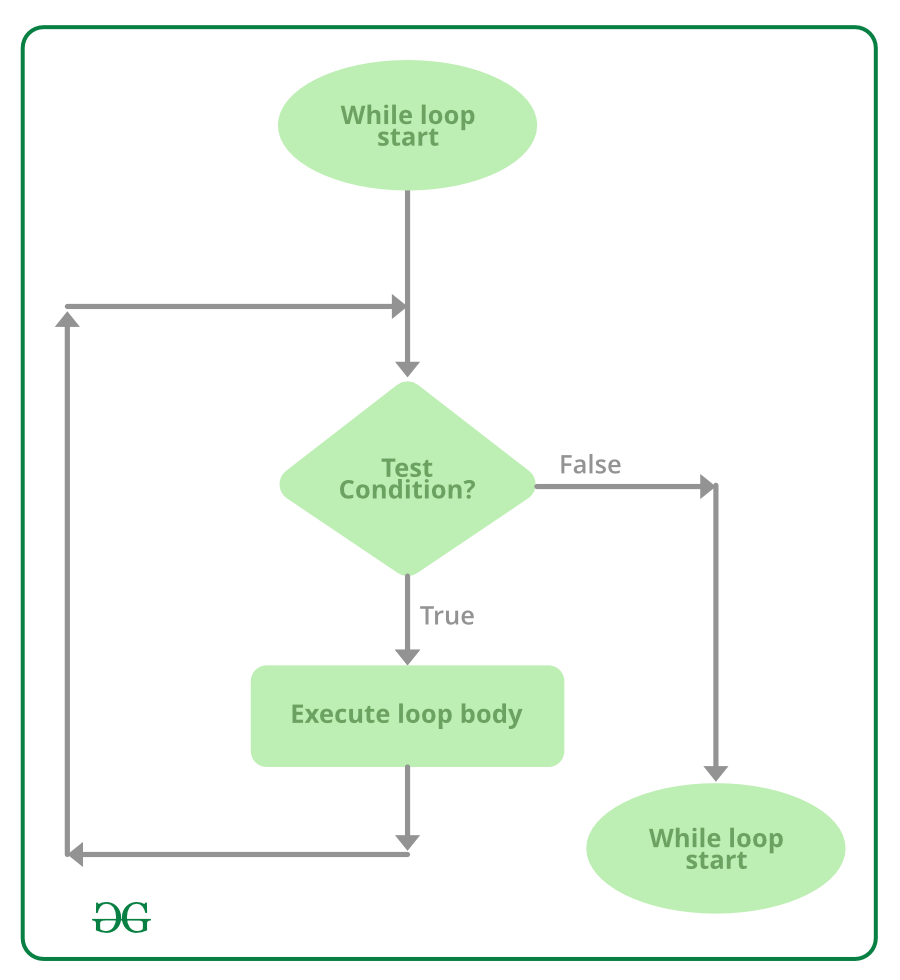# while Loop in C#

• Last Updated : 25 Aug, 2020

Looping in a programming language is a way to execute a statement or a set of statements multiple number of times depending on the result of the condition to be evaluated. while loop is an Entry Controlled Loop in C#.

The test condition is given in the beginning of the loop and all statements are executed till the given boolean condition satisfies, when the condition becomes false, the control will be out from the while loop.

Syntax:

```while (boolean condition)
{
loop statements...
}
```

Flowchart:Example 1:

 `// C# program to illustrate while loop``using` `System;`` ` `class` `whileLoopDemo {``    ``public` `static` `void` `Main()``    ``{``        ``int` `x = 1;`` ` `        ``// Exit when x becomes greater than 4``        ``while` `(x <= 4) {``            ``Console.WriteLine(``"GeeksforGeeks"``);`` ` `            ``// Increment the value of x for``            ``// next iteration``            ``x++;``        ``}``    ``}``}`

Output:

```GeeksforGeeks
GeeksforGeeks
GeeksforGeeks
GeeksforGeeks
```

Example 2:

 `// C# program to illustrate while loop``using` `System;`` ` `class` `whileLoopDemo {``    ``public` `static` `void` `Main()``    ``{``        ``int` `x = 10;`` ` `        ``// Exit when x becomes less than 5``        ``while` `(x > 4) {``            ``Console.WriteLine(``"Value "` `+ x);`` ` `            ``// Decrement the value of x for``            ``// next iteration``            ``x--;``        ``}``    ``}``}`

Output:

```Value 10
Value 9
Value 8
Value 7
Value 6
Value 5
```

My Personal Notes arrow_drop_up